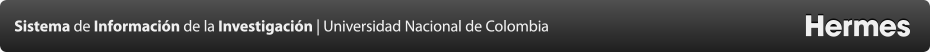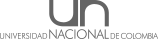Grupos de investigación
Algoritmos y combinatoria (algos­un)
Presentacion
The Research Group on ALGORITHMS AND COMBINATORICS (ALGOS), is a research group that supports undergraduate, master's and doctorate in Systems Engineering and Computer Science and Industrial Engineering, Faculty of Engineering of the National University of Colombia in Bogotá. The scope ALGOS research is the basic and applied algorithmics study: the science and art of designing algorithms for solving combinatorial problems or continuous problems whose solution can be approximated discretely that appear systematically in various areas of science. Group members work on: analysis and design of data structures, analysis and design of algorithms, parallel algorithms,, evolutionary algorithms, approximate algorithms, online algorithms, randomized algorithms, probabilistic analysis of algorithms, probabilistic models in computing, combinatorial analysis, probabilistic combinatorics, computational game theory and graph theory. Group members also work developing algorithms that solve efficiently and effectively problems in applied areas as: computational finance, algorithmic trading, FinTech, data science, machine learning, bioinformatics, computational neuroscience, optimization, fuzzy optimization, approximated search, simulation, classification systems and data management, encryption of information, numerical analysis, numerical calculation and the teaching-learning of computational thinking and programming. The group has a Research Subgroup on ALGORITHMIC TRADING, COMPUTATIONAL FINANCES AND FINTECH (ALGOS-ALGOTRADE). Members of this subgroup work on: computational finance, algorithmic trading, FinTech, computational markets, design of market mechanisms, design of algorithmic trading strategies, design of trading strategies based on data science, machine learning and computational intelligence, design of trading strategies based on computational game theory,
Líder
Sedes
Bogotá
Otras Sedes
• Bogotá
• Medellín
Dependencias
2- FACULTAD DE INGENIERÍA
Planes de estudio
• MAESTRIA EN INGENIERIA - AUTOMATIZACION INDUSTRIAL
• INGENIERÍA DE SISTEMAS Y COMPUTACIÓN - SEDE BOGOTÁ - AC 033
• INGENIERIA INDUSTRIAL
• DOCTORADO EN INGENIERIA - SISTEMAS Y COMPUTACION
• DOCTORADO EN INGENIERÍA - INDUSTRIA Y ORGANIZACIONES
• CIENCIAS DE LA COMPUTACIÓN
• MAESTRIA EN INGENIERIA - INGENIERIA DE SISTEMAS Y COMPUTACIÓN
Agendas de conocimiento
Tecnologías de la Información y Comunicaciones
Agendas del conocimiento secundarias
• Desarrollo Organizacional Económico e Industrial
Áreas OCDE
Ingeniería y tecnología - Ingenierías eléctrica, electrónica e informática
Lineas de investigación
• ALGORITMOS Y COMPLEJIDAD COMPUTACIONAL
Enfoque estratégico
The Research Group on ALGORITHMS AND COMBINATORICS (ALGOS), is a research group that supports undergraduate, master's and doctorate in Systems Engineering and Computer Science and Industrial Engineering, Faculty of Engineering of the National University of Colombia in Bogotá. The scope ALGOS research is the basic and applied algorithmics study: the science and art of designing algorithms for solving combinatorial problems or continuous problems whose solution can be approximated discretely that appear systematically in various areas of science. Group members work on: analysis and design of data structures, analysis and design of algorithms, parallel algorithms,, evolutionary algorithms, approximate algorithms, online algorithms, randomized algorithms, probabilistic analysis of algorithms, probabilistic models in computing, combinatorial analysis, probabilistic combinatorics, computational game theory and graph theory. Group members also work developing algorithms that solve efficiently and effectively problems in applied areas as: computational finance, algorithmic trading, FinTech, data science, machine learning, bioinformatics, computational neuroscience, optimization, fuzzy optimization, approximated search, simulation, classification systems and data management, encryption of information, numerical analysis, numerical calculation and the teaching-learning of computational thinking and programming. The group has a Research Subgroup on ALGORITHMIC TRADING, COMPUTATIONAL FINANCES AND FINTECH (ALGOS-ALGOTRADE). Members of this subgroup work on: computational finance, algorithmic trading, FinTech, computational markets, design of market mechanisms, design of algorithmic trading strategies, design of trading strategies based on data science, machine learning and computational intelligence, design of trading strategies based on computational game theory,
Prioridades de investigación
The Research Group on ALGORITHMS AND COMBINATORICS (ALGOS), is a research group that supports undergraduate, master's and doctorate in Systems Engineering and Computer Science and Industrial Engineering, Faculty of Engineering of the National University of Colombia in Bogotá. The scope ALGOS research is the basic and applied algorithmics study: the science and art of designing algorithms for solving combinatorial problems or continuous problems whose solution can be approximated discretely that appear systematically in various areas of science. Group members work on: analysis and design of data structures, analysis and design of algorithms, parallel algorithms,, evolutionary algorithms, approximate algorithms, online algorithms, randomized algorithms, probabilistic analysis of algorithms, probabilistic models in computing, combinatorial analysis, probabilistic combinatorics, computational game theory and graph theory. Group members also work developing algorithms that solve efficiently and effectively problems in applied areas as: computational finance, algorithmic trading, FinTech, data science, machine learning, bioinformatics, computational neuroscience, optimization, fuzzy optimization, approximated search, simulation, classification systems and data management, encryption of information, numerical analysis, numerical calculation and the teaching-learning of computational thinking and programming. The group has a Research Subgroup on ALGORITHMIC TRADING, COMPUTATIONAL FINANCES AND FINTECH (ALGOS-ALGOTRADE). Members of this subgroup work on: computational finance, algorithmic trading, FinTech, computational markets, design of market mechanisms, design of algorithmic trading strategies, design of trading strategies based on data science, machine learning and computational intelligence, design of trading strategies based on computational game theory,
Perspectiva interdisciplinaria
Trabajmos con el Grupo Odeon del Externado y con Quantopian a nivel intrenacional
Integrantes
Proyectos
Productos
• A Complex Network Approach to Identify Potential Financial Scandals: The Colombian Market Case (Artículos sometidos a revisión en revista indexada.)
• Learning of natural trading strategies on foreign exchange high-frequency market data using dynamic Bayesian networks (Capítulo en libro que presente resultados de la investigación)
• Designing, Implementing and Testing an Automated Trading Strategy Based on Dynamic Bayesian Networks, the Limit Order Book Information, and the Random Entry Protocol. (Capítulo en libro que presente resultados de la investigación)
• AN HYBRID EVOLUTIONARY ALGORITHM FOR THE VEHICLE ROUTING PROBLEMA WITH STOCHASTIC DEMANS (DIRECCIÓN DE TESIS)
• DISEÑO Y EVALUACIÓN DE UN NUEVO MODELO DE GENERACIÓN DE SECUENCIAS PSEUDOALEATORIAS USANDO AUTÓMATAS CELULARES Y SU APLICABILIDAD EN CRIPTOGRAFÍA ACORDE A LOS ESTÁNDARES ACTUALES (DIRECCIÓN DE TESIS)
• HIGH FREQUENCY EXCHANGE RATE PREDICTION USING DYNAMIC BAYESIAN NETWORKS OVER THE LIMIT ORDER BOOK INFORMATION (DIRECCIÓN DE TESIS)
• FUZZY LINEAR PROGRAMMING WITH INTERVAL TYPE-2 FUZZY CONSTRAINTS (DIRECCIÓN DE TESIS)
• COMPUTATIONAL VISUAL ANALYSIS OF THE ORDER BOOK DYNAMICS FOR CREATING HIGH-FREQUENCY FOREIGN EXCHANGE TRADING STRATEGIES. (PONENCIAS EN EVENTOS ESPECIALIZADOS)
• HACIA LA OPTIMIZACIÓN USANDO COMPUTACIÓN CON PALABRAS (PUBLICACIÓN EN ARTÍCULO DE REVISTA)
• A METHOD FOR SOLVING LINEAR PROGRAMMING MODELS WITH INTERVAL TYPE-2 FUZZY CONSTRAINTS (PUBLICACIÓN EN ARTÍCULO DE REVISTA)
• Deep Learning Neural Network based Algorithmic Trading Strategy for Colombian Financial Market using Tick-by-Tick and Order Book Data (Tesis doctoral)